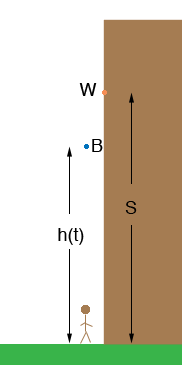SEARCH HOMEMath Central Quandaries & QueriesHow do I solve this? The height h (in feet) of an object that is dropped from the height of s feet is given by the formula h = s - 16t^2 , where t is the time the object has been falling. A 6 foot tall woman on a sidewalk looks directly overhead and sees a window washer drop a bottle from the 6 story. How long does she have to get out of the way? Round to the nearest tenth. (A story is 12 feet.)Hi Sharon,

I am a very visual person so I drew a diagram. W is the position of the window washer and B is the position of the bottle t seconds after it is dropped. I wrote the height of the bottle off the ground as h(t) rather than h since it is a function of t.Since the window washer is 6 stories up and each story is 12 feet, s = 612 = 72 feet. The woman is 6 feet tall so if she stays put the bottle will strike the top of her head when h(t) = 6 feet. Substitute h(t) = 6 feet into the expression

h(t) = s - 16 t2

and solve for t.

PennyMath Central is supported by the University of Regina and The Pacific Institute for the Mathematical Sciences.